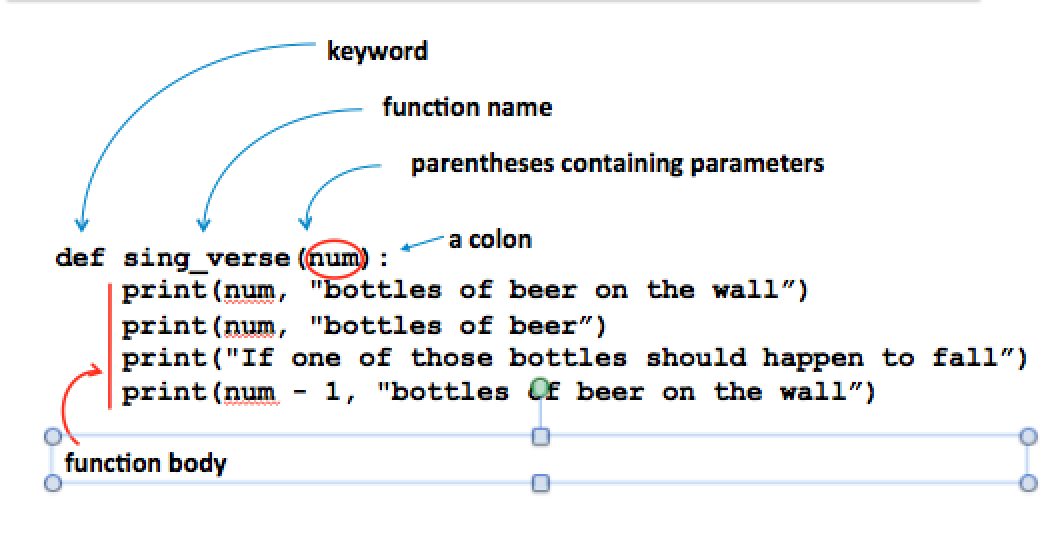# Lab 4 - Let’s get Functional!¶

In this short lab, we will be reviewing how to write our own functions in Python. The exercises are based on Chapter 7 of There Are Eels in My Hovercraft. If you have not read that chapter yet, you should review it now and try out the code examples.

## Part 1: Writing Our Own Functions¶

Let’s look at structure of a function. Here is an illustration from Chapter 7 showing the basic structure of any function:A function is just a block of code (the function body) with a name attached to it (such as sing_verse) so that we can call the function to perform the block of statements. The function definition doesn’t do anything until we call it.

Step through the code below using codelens. Notice that the first statement executed is sing_verse(99). Calling the function causes the interpreter to go and execute the body of the function. When it reaches the end of the function body, it returns to point where it was called, and proceeds with the next line of code.

### Function parameters¶

In order to correctly print a verse of the song, the function needs a piece of information, namely, the number of bottles currently on the wall. In order to provide that information, we use a special variable called a parameter. In this case, the parameter is called num. This variable is defined in the parentheses of the function definition.

When the function is called, we have to supply a value for that variable, such as the number 99 in the line sing_verse(99).

It is possible for a function to have no parameters like the one below. Notice we still need the empty parentheses!

### Functions With Multiple Parameters¶

When there is more than one parameter, they are separated by a comma. Let’s write a function that takes two numbers as parameters and prints quotient and remainder.

sc-1-5: Suppose you are defining a function area that prints the area of a square. What should be the first line of the function definition?
• (A) def area(length, width):
• You don't need two parameters for a square, width and height are the same
• (B) def area(side):
• Good
• Normally the word 'radius' refers to a circle
• (D) def area(length, width, height):
• You might need three parameters for a 3-d solid

### Checkpoint 1¶

In Wing 101, create three files as follows.

1. Create a file greetings.py and in it write a function that prints Birthday Wishes to your friend. The function takes your friend’s name as parameter and prints Happy Birthday, *name*!. Then, create a separate file **test_functions.py that tests the function by print birthday greetings for all your friends who are celebrating a birthday this month. (Be sure to include Steve, Kate, and Preeti!) Remember you’ll need an import statement (see the example in Chapter 7).
2. Make a new file, called hypotenuse.py. For this checkpoint, write a Python function that, given the lengths of the two legs of a right triangle, prints the length of the hypotenuse. (Remember you can get the square root using math.sqrt().) Add some test cases to your test_functions.py script.

### Value Returning Functions¶

In the examples above, we write a function to produce some output. By creating a function, we don’t need to copy and paste the code when we need to the same task more than once - we can just call the function again.

However, we don’t always want a function to produce output: sometimes we just want it to compute a value and give the value back to us, so that we can use it later as we choose. This situation, in fact is much more common. Most of the Python library functions don’t produce output. For example, in the script hypotenuse.py, you called math.sqrt to compute a square root for you. It would be pretty weird, and would mess up your code, if it also printed its own output!

To make a function give you a value, it needs a return statement. As an example, here’s a function that computes the area of a rectangle.

In our previous examples, we would have a line of code such as

do_division(12, 5)


Notice we are using the function call as a statement: its an instruction to perform some action. By contrast, when we write the line

answer = find_area(10, 20)


the call find_area(10, 20) is an expression with value 200. It doesn’t “do” anything, it just “is”. If you call the function, but you don’t do anything with the returned value, it will seem to have no effect. Try this:

You might also have the opposite problem. What happens if you take a function that doesn’t have a return statement, and try to use its value? Try this.

What is printed by this code? Choose the best answer below.

def sub(x, y)
result = x - y

x = 4
y = 10
print(sub(x, y))

sc-1-6: (See the code above.)
• (A) 6
• The function has no return value
• (B) -6
• The function has no return value
• (C) Error, since x is less than y
• Actually, 4 - 10 is -6
• (D) The value None
• Since there is no return value, we get the special value None

If you try to examine the value of a function that has no return value, you’ll get the special Python value None. When you see “None” in your output, it is a sign that your function isn’t actually returning a value, but you’re trying to use the value anyway.

### Checkpoint 2¶

Donuts are .75 each or 8.00 per dozen. Write a module donuts.py with a function called donut_break that has one parameter, the number of donuts, and returns the cost for the donuts.

Write a separate script to test your function using several different values. Calculate what the answers should be and make sure your function is correct.